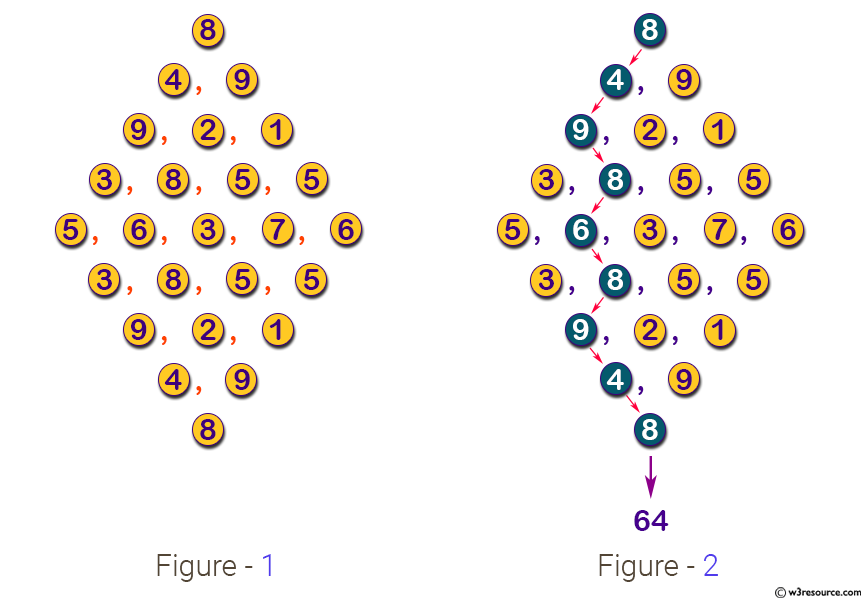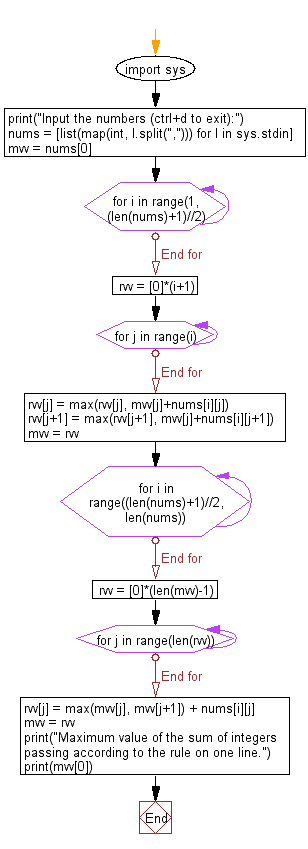﻿ Python: Compute the maximum value of the sum of the passing integers - w3resource# Python: Compute the maximum value of the sum of the passing integers

## Python Basic - 1: Exercise-61 with SolutionArrange integers (0 to 99) as narrow hilltop, as illustrated in Figure 1. Reading such data representing huge, when starting from the top and proceeding according to the next rule to the bottom. Write a Python program that compute the maximum value of the sum of the passing integers according to the following rules.

At each step, you can go to the lower left diagonal or the lower right diagonal. For example, in the example of FIG. 1, as shown in FIG. 2, when 8,4,9,8,6,8,9,4,8 is selected and passed, the sum is 64 (8 + 4 + 9) + 8 + 6 + 8 + 9 + 4 + 8 = 64)

Input:
A series of integers separated by commas are given in diamonds. No spaces are included in each line. The input example corresponds to Figure 1. The number of lines of data is less than 100 lines.

Ref: https://bit.ly/3ct00TY

Sample Solution:

Python Code:

``````import sys
print("Input the numbers (ctrl+d to exit):")
nums = [list(map(int, l.split(","))) for l in sys.stdin]
mvv = nums

for i in range(1, (len(nums)+1)//2):
rvv = *(i+1)
for j in range(i):
rvv[j] = max(rvv[j], mvv[j]+nums[i][j])
rvv[j+1] = max(rvv[j+1], mvv[j]+nums[i][j+1])
mvv = rvv

for i in range((len(nums)+1)//2, len(nums)):
rvv = *(len(mvv)-1)
for j in range(len(rvv)):
rvv[j] = max(mvv[j], mvv[j+1]) + nums[i][j]
mvv = rvv
print("Maximum value of the sum of integers passing according to the rule on one line.")
print(mvv)
``````

Sample Output:

```Input the numbers (ctrl+d to exit):
8
4, 9
9, 2, 1
3, 8, 5, 5
5, 6, 3, 7, 6
3, 8, 5, 5
9, 2, 1
4, 9
8
Maximum value of the sum of integers passing according to the rule on one line.
64
```

Flowchart:Python Code Editor:

Have another way to solve this solution? Contribute your code (and comments) through Disqus.

What is the difficulty level of this exercise?

Test your Programming skills with w3resource's quiz.

﻿

## Python: Tips of the Day

How to make a flat list out of list of lists?

Given a list of lists l

```flat_list = [item for sublist in l for item in sublist]
```

which means:

```flat_list = []
for sublist in l:
for item in sublist:
flat_list.append(item)
```

is faster than the shortcuts posted so far. (l is the list to flatten.) Here is the corresponding function:

flatten = lambda l: [item for sublist in l for item in sublist]

As evidence, you can use the timeit module in the standard library:

```\$ python -mtimeit -s'l=[[1,2,3],[4,5,6], , [8,9]]*99' '[item for sublist in l for item in sublist]'
10000 loops, best of 3: 143 usec per loop
\$ python -mtimeit -s'l=[[1,2,3],[4,5,6], , [8,9]]*99' 'sum(l, [])'
1000 loops, best of 3: 969 usec per loop
\$ python -mtimeit -s'l=[[1,2,3],[4,5,6], , [8,9]]*99' 'reduce(lambda x,y: x+y,l)'
1000 loops, best of 3: 1.1 msec per loop
```

Explanation: the shortcuts based on + (including the implied use in sum) are, of necessity, O(L**2) when there are L sublists -- as the intermediate result list keeps getting longer, at each step a new intermediate result list object gets allocated, and all the items in the previous intermediate result must be copied over (as well as a few new ones added at the end). So, for simplicity and without actual loss of generality, say you have L sublists of I items each: the first I items are copied back and forth L-1 times, the second I items L-2 times, and so on; total number of copies is I times the sum of x for x from 1 to L excluded, i.e., I * (L**2)/2.

The list comprehension just generates one list, once, and copies each item over (from its original place of residence to the result list) also exactly once.

Ref: https://bit.ly/3dKsNTR